Finding AP

Chapter 5 Class 10 Arithmetic Progressions (Term 2)
Concept wise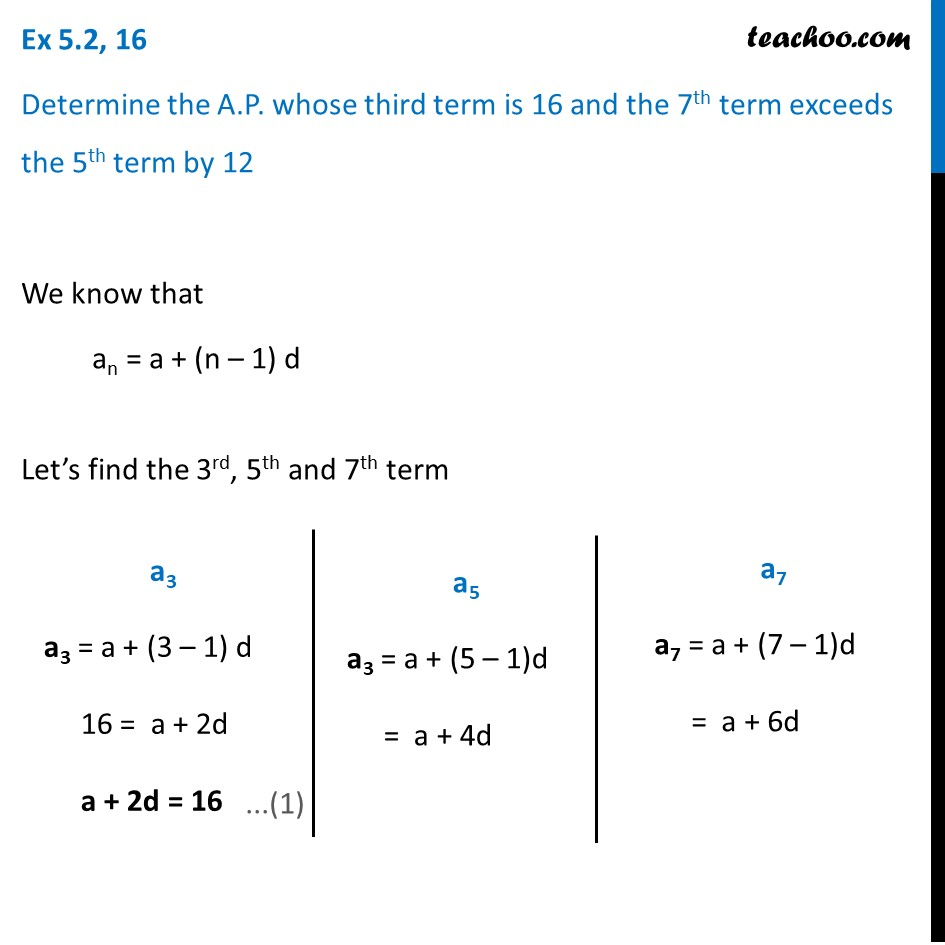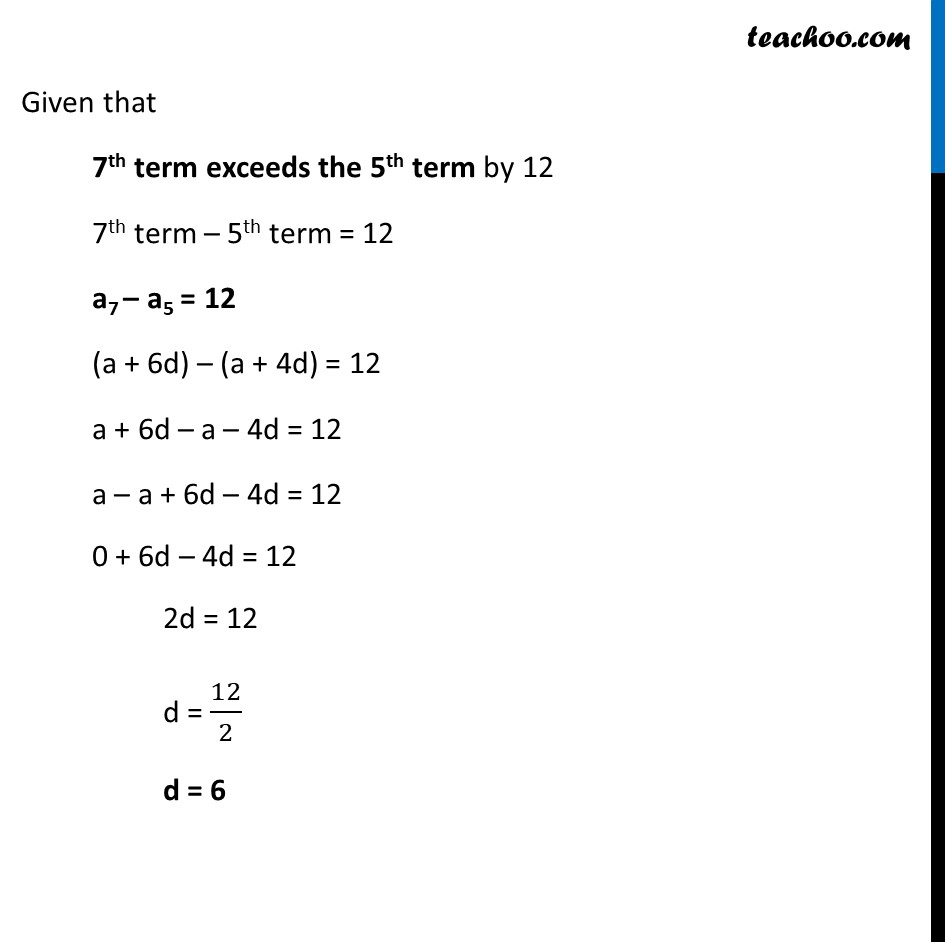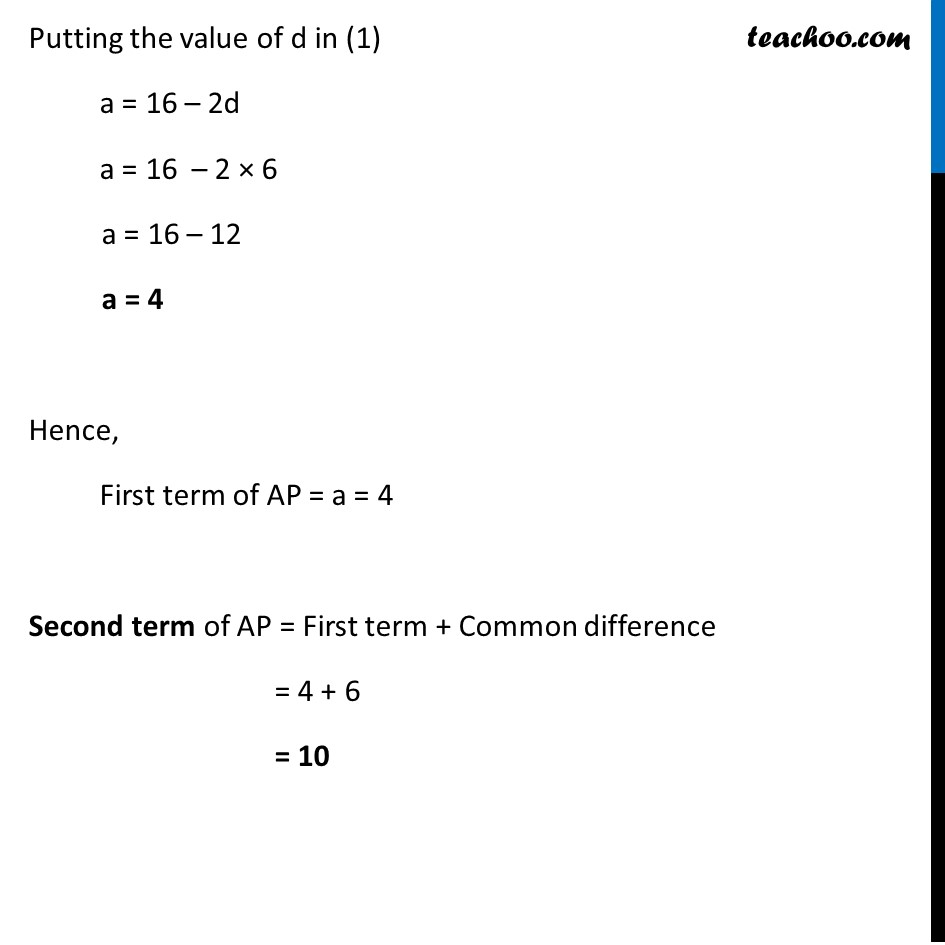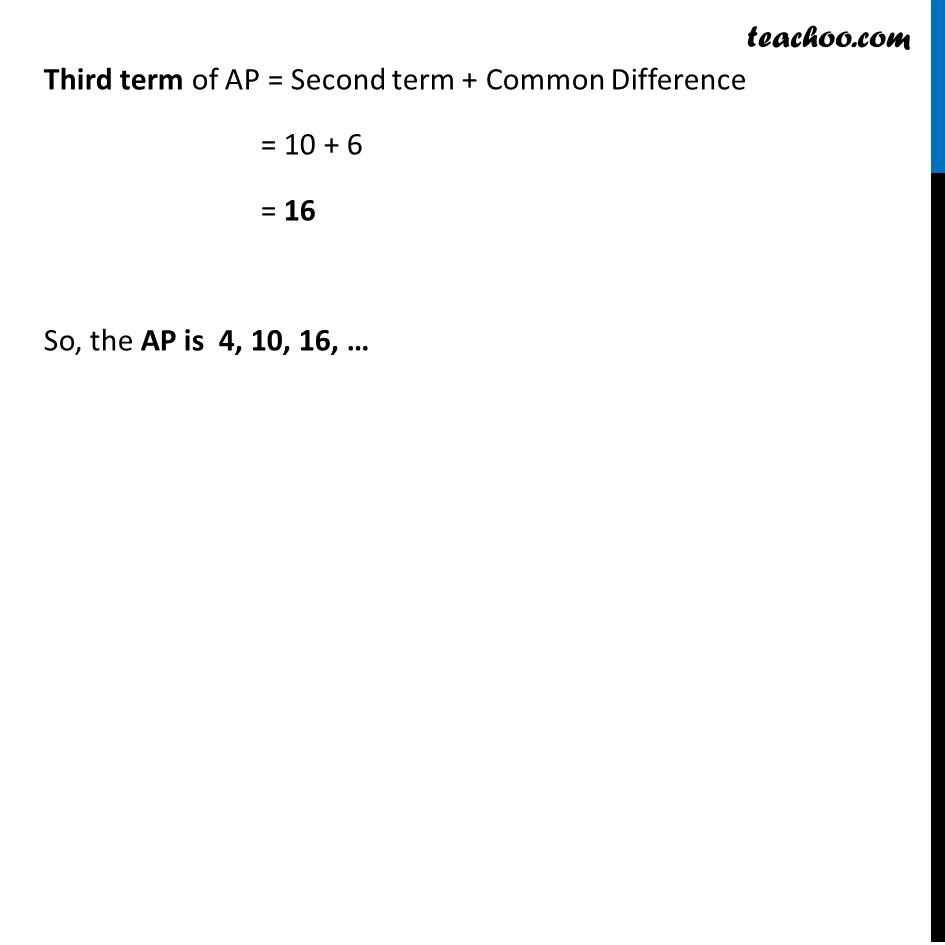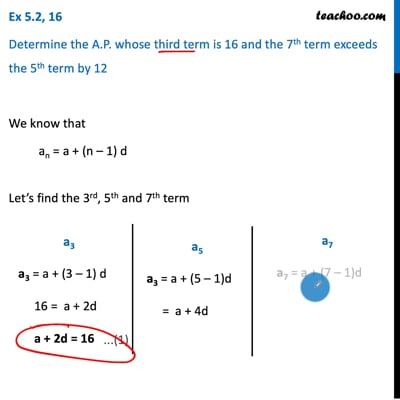This video is only available for Teachoo black users

### Transcript

Ex 5.2, 16 Determine the A.P. whose third term is 16 and the 7th term exceeds the 5th term by 12 We know that an = a + (n – 1) d Let’s find the 3rd, 5th and 7th term a3 a3 = a + (3 – 1) d 16 = a + 2d a + 2d = 16 a5 a3 = a + (5 – 1)d = a + 4d a7 a7 = a + (7 – 1)d = a + 6d Given that 7th term exceeds the 5th term by 12 7th term – 5th term = 12 a7 – a5 = 12 (a + 6d) – (a + 4d) = 12 a + 6d – a – 4d = 12 a – a + 6d – 4d = 12 0 + 6d – 4d = 12 2d = 12 d = 12/2 d = 6 Putting the value of d in (1) a = 16 – 2d a = 16 – 2 × 6 a = 16 – 12 a = 4 Hence, First term of AP = a = 4 Second term of AP = First term + Common difference = 4 + 6 = 10 Third term of AP = Second term + Common Difference = 10 + 6 = 16 So, the AP is 4, 10, 16, …